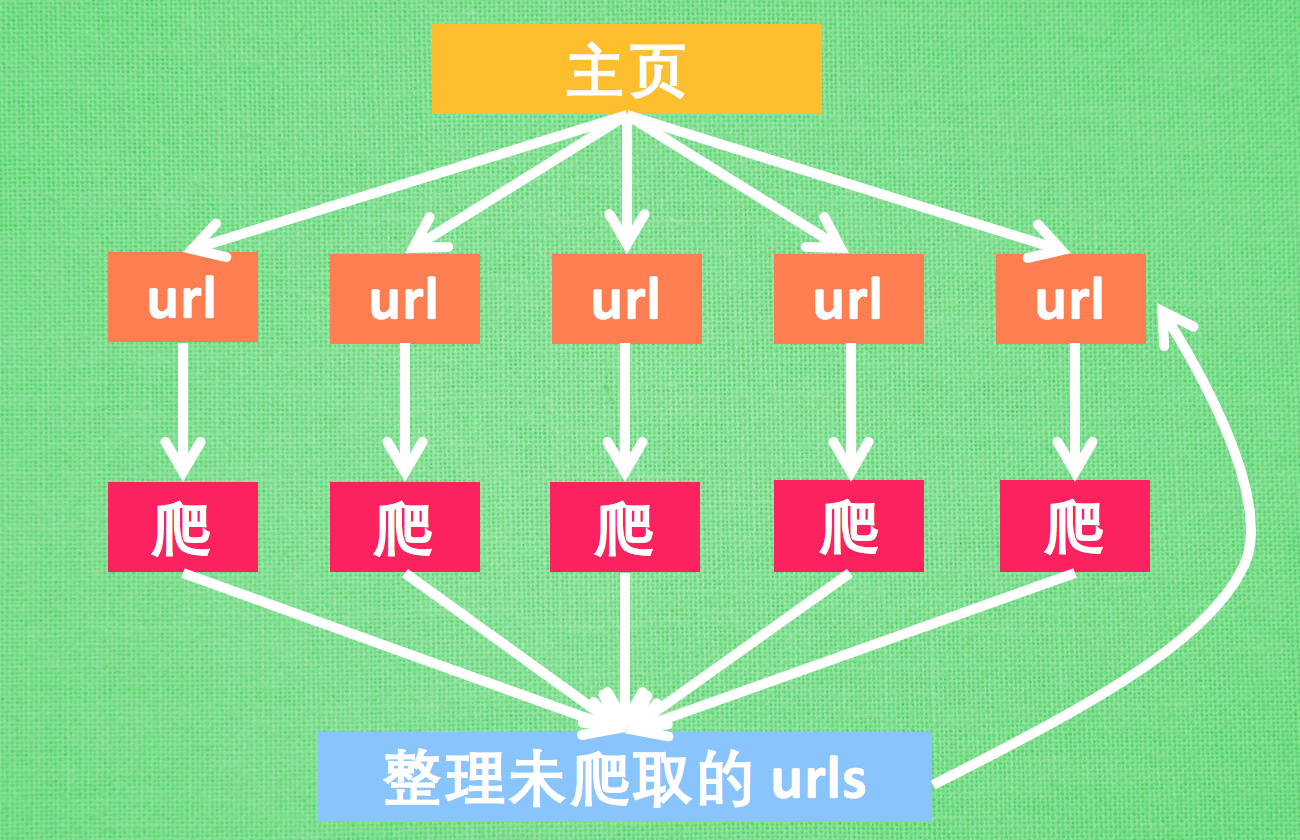``````if __name__ == __main__
``````

(Python中的multiprocess提供了Process类，实现进程相关的功能。但是它基于fork机制，因此不被windows平台支持。想要在windows中运行，必须使用该的方式)，但是我有另一种方法在使用线程池的时候可以不使用name_mian，最下面说。

# 案例一 基础用法

``````import sys
import multiprocessing
sys.setdefaultencoding('utf-8')
def fun(i):
print sys.path
print sys.version_info
print sys.platform
print sys.long_info

if __name__ == '__main__':
m = multiprocessing.Process(target=fun,args=(1,))
m.start()
``````

``````['E:\\python27\\python study', 'E:\\python27', 'C:\\windows\\SYSTEM32\\python27.zip', 'F:\\Python27\\DLLs', 'F:\\Python27\\lib', 'F:\\Python27\\lib\\plat-win', 'F:\\Python27\\lib\\lib-tk', 'F:\\Python27', 'F:\\Python27\\lib\\site-packages', 'F:\\Python27\\lib\\site-packages\\certifi-2017.7.27.1-py2.7.egg', 'F:\\Python27\\lib\\site-packages\\idna-2.6-py2.7.egg', 'F:\\Python27\\lib\\site-packages\\pypiwin32-219-py2.7-win-amd64.egg', 'F:\\Python27\\lib\\site-packages\\future-0.16.0-py2.7.egg', 'F:\\Python27\\lib\\site-packages\\dis3-0.1.1-py2.7.egg', 'F:\\Python27\\lib\\site-packages\\macholib-1.8-py2.7.egg', 'F:\\Python27\\lib\\site-packages\\pefile-2017.9.3-py2.7.egg', 'F:\\Python27\\lib\\site-packages\\altgraph-0.14-py2.7.egg', 'F:\\Python27\\lib\\site-packages\\beautifulsoup4-4.6.0-py2.7.egg', 'F:\\Python27\\lib\\site-packages\\chardet-3.0.4-py2.7.egg']
sys.version_info(major=2, minor=7, micro=14, releaselevel='final', serial=0)
win32
sys.long_info(bits_per_digit=30, sizeof_digit=4)
``````

# 案例二 数据通信

ipc：就是进程间的通信模式，常用的一半是socke，rpc，pipe和消息队列等。

## 使用Array共享数据

``````'c': ctypes.c_char, 'u': ctypes.c_wchar,
'b': ctypes.c_byte, 'B': ctypes.c_ubyte,
'h': ctypes.c_short, 'H': ctypes.c_ushort,
'i': ctypes.c_int, 'I': ctypes.c_uint,
'l': ctypes.c_long, 'L': ctypes.c_ulong,
'f': ctypes.c_float, 'd': ctypes.c_double
``````

``````from multiprocessing import Process
from multiprocessing import Array

def func(i,temp):
temp += 100
print("进程%s " % i, ' 修改数组第一个元素后----->', temp)

if __name__ == '__main__':
temp = Array('i', [1, 2, 3, 4])
for i in range(10):
p = Process(target=func, args=(i, temp))
p.start()
``````

``````进程2   修改数组第一个元素后-----> 101

``````

## 使用Manager共享数据

``````from multiprocessing import Process
from multiprocessing import Manager

def func(i, dic):
dic["num"] = 100+i
print(dic.items())

if __name__ == '__main__':
dic = Manager().dict()
for i in range(10):
p = Process(target=func, args=(i, dic))
p.start()
p.join()
``````

## 使用queues的Queue类共享数据

multiprocessing是一个包，它内部有一个queues模块，提供了一个Queue队列类，可以实现进程间的数据共享，如下例所示：

``````import multiprocessing
from multiprocessing import Process
from multiprocessing import queues

def func(i, q):
ret = q.get()
print("进程%s从队列里获取了一个%s，然后又向队列里放入了一个%s" % (i, ret, i))
q.put(i)

if __name__ == "__main__":
lis = queues.Queue(20, ctx=multiprocessing)
lis.put(0)
for i in range(10):
p = Process(target=func, args=(i, lis,))
p.start()
``````

``````进程1从队列里获取了一个0，然后又向队列里放入了一个1

``````

## 使用pipe实现进程间通信

pipe只能适用于两个进程间通信，queue则没这个限制，他有两个方法

``````receive_pi = Pipe()
# 定义变量，用来获取数据
send_pi = Pipe()
# 用来发送数据
``````

``````from multiprocessing import Pipe,Process
import time
def produce(pipe):
pipe.send('666')
time.sleep(1)
def consumer(pipe):
print(pipe.recv())
# 有些类似socket的recv方法
if __name__ == '__main__':
send_pi,recv_pi = Pipe()
my_pro = Process(target=produce,args=(send_pi,))
my_con = Process(target=consumer,args=(recv_pi,))
my_pro.start()
my_con.start()
my_pro.join()
my_con.join()
``````

pipe相当于queue的一个子集，只能服务两个进程，pipe的性能高于queue。

# 案例三 进程锁

``````from multiprocessing import Process
from multiprocessing import Array
from multiprocessing import RLock, Lock, Event, Condition, Semaphore
import time

def func(i,lis,lc):
lc.acquire()
lis = lis - 1
time.sleep(1)
print('say hi', lis)
lc.release()

if __name__ == "__main__":
array = Array('i', 1)
array = 10
lock = RLock()
for i in range(10):
p = Process(target=func, args=(i, array, lock))
p.start()
``````

``````say hi 9
say hi 8
say hi 7
say hi 6
say hi 5
say hi 4
say hi 3
say hi 2
say hi 1
say hi 0
``````

# 案例四 进程池

from multiprocessing import Pool导入就行，非常容易使用的。进程池内部维护了一个进程序列，需要时就去进程池中拿取一个进程，如果进程池序列中没有可供使用的进程，那么程序就会等待，直到进程池中有可用进程为止。

1. apply() 同步执行（串行）
2. apply_async() 异步执行（并行）
3. terminate() 立刻关闭进程池
4. join() 主进程等待所有子进程执行完毕。必须在close或terminate()之后。
5. close() 等待所有进程结束后，才关闭进程池。

``````from multiprocessing import Pool
import time
def func(args):
time.sleep(1)
print("正在执行进程 ", args)
if __name__ == '__main__':
p = Pool(5)     # 创建一个包含5个进程的进程池
for i in range(30):
# 有30个任务
p.apply_async(func=func, args=(i,))
# 异步执行，并发。这里不用target，要用func
p.close()           # 等子进程执行完毕后关闭进程池
# time.sleep(2)
# p.terminate()     # 立刻关闭进程池
p.join()
``````

from multiprocessing.dummy import Pool as ThreadPool 是多线程进程池，绑定一个cpu核心。from multiprocessing import Pool多进程，运行于多个cpu核心。multiprocessing 是多进程模块， 而multiprocessing.dummy是以相同API实现的多线程模块。

``````from multiprocessing.dummy import Pool as tp
def fun(i):
print i+i+i+i

list_i=[range(100)]

px = tp(processes=8)
# 开启8个线程池
px.map(fun,list_i)
px.close()
px.join()
``````

# 案例五 爬虫进程池

## 我们的分布式爬虫``````import multiprocessing as mp
import time
from urllib.request import urlopen, urljoin
from bs4 import BeautifulSoup
import re

# base_url = "http://127.0.0.1:4000/"
base_url = 'https://morvanzhou.github.io/'
``````

``````def crawl(url):
response = urlopen(url)

def parse(html):
soup = BeautifulSoup(html, 'lxml')
urls = soup.find_all('a', {"href": re.compile('^/.+?/\$')})
title = soup.find('h1').get_text().strip()
page_urls = set([urljoin(base_url, url['href']) for url in urls])   # 去重
url = soup.find('meta', {'property': "og:url"})['content']
return title, page_urls, url
``````

``````unseen = set([base_url,])
seen = set()
``````

## 测试普通爬法

``````# DON'T OVER CRAWL THE WEBSITE OR YOU MAY NEVER VISIT AGAIN
if base_url != "http://127.0.0.1:4000/":
restricted_crawl = True
else:
restricted_crawl = False

while len(unseen) != 0:                 # still get some url to visit
if restricted_crawl and len(seen) >= 20:
break
htmls = [crawl(url) for url in unseen]
results = [parse(html) for html in htmls]

seen.update(unseen)         # seen the crawled
unseen.clear()              # nothing unseen

for title, page_urls, url in results:
unseen.update(page_urls - seen)     # get new url to crawl
``````

## 测试分布式爬法

``````pool = mp.Pool(4)
while len(unseen) != 0:
# htmls = [crawl(url) for url in unseen]
# --->
crawl_jobs = [pool.apply_async(crawl, args=(url,)) for url in unseen]
htmls = [j.get() for j in crawl_jobs]

# results = [parse(html) for html in htmls]
# --->
parse_jobs = [pool.apply_async(parse, args=(html,)) for html in htmls]
results = [j.get() for j in parse_jobs]
``````

# 各模块作用

## Process介绍

1. Process([group [, target [, name [, args [, kwargs]]]]])
2. group: 线程组，目前还没有实现，库引用中提示必须是None；
3. target: 要执行的方法；
4. name: 进程名；
5. args/kwargs: 要传入方法的参数。

1. is_alive()：返回进程是否在运行。
2. join([timeout])：阻塞当前上下文环境的进程程，直到调用此方法的进程终止或到达指定的3. timeout（可选参数）。
3. start()：进程准备就绪，等待CPU调度。
4. run()：strat()调用run方法，如果实例进程时未制定传入target，这star执行t默认run()方法。
5. terminate()：不管任务是否完成，立即停止工作进程。

1. authkey
2. daemon：和线程的setDeamon功能一样（将父进程设置为守护进程，当父进程结束时，子进程也结束）。
3. exitcode(进程在运行时为None、如果为–N，表示被信号N结束）。
4. name：进程名字。
5. pid：进程号。

## Pool介绍

Multiprocessing.Pool可以提供指定数量的进程供用户调用，当有新的请求提交到pool中时，如果池还没有满，那么就会创建一个新的进程用来执行该请求；但如果池中的进程数已经达到规定最大值，那么该请求就会等待，直到池中有进程结束，才会创建新的进程来执行它。在共享资源时，只能使用Multiprocessing.Manager类，而不能使用Queue或者Array。Pool类用于需要执行的目标很多，而手动限制进程数量又太繁琐时，如果目标少且不用控制进程数量则可以用Process类。

1. Pool([processes[, initializer[, initargs[, maxtasksperchild[, context]]]]])
2. processes ：使用的工作进程的数量，如果processes是None那么使用 os.cpu_count()返回的数量。
3. initializer： 如果initializer是None，那么每一个工作进程在开始的时候会调用initializer(*initargs)。
5. context: 用在制定工作进程启动时的上下文，一般使用 multiprocessing.Pool() 或者一个context对象的Pool()方法来创建一个池，两种方法都适当的设置了context。

1. apply_async(func[, args[, kwds[, callback]]]) 它是非阻塞。
2. apply(func[, args[, kwds]])是阻塞的
3. close() 关闭pool，使其不在接受新的任务。
4. terminate() 关闭pool，结束工作进程，不在处理未完成的任务。
5. join() 主进程阻塞，等待子进程的退出， join方法要在close或terminate之后使用。

Pool使用方法

Pool+map函数

``````from multiprocessing import Pool
def test(i):
print i
if __name__=="__main__":
lists=[1,2,3]
pool=Pool(processes=2) #定义最大的进程数
pool.map(test,lists)        #p必须是一个可迭代变量。
pool.close()
pool.join()
``````

``````from multiprocessing import Pool
def test(i):
print i
if __name__=="__main__":
pool = Pool(processes=10)
for i  in xrange(500):
'''
For循环中执行步骤：
（1）循环遍历，将500个子进程添加到进程池（相对父进程会阻塞）
（2）每次执行10个子进程，等一个子进程执行完后，立马启动新的子进程。（相对父进程不阻塞）

apply_async为异步进程池写法。
异步指的是启动子进程的过程，与父进程本身的执行（print）是异步的，而For循环中往进程池添加子进程的过程，与父进程本身的执行却是同步的。
'''
pool.apply_async(test, args=(i,)) #维持执行的进程总数为10，当一个进程执行完后启动一个新进程.
print “test”
pool.close()
pool.join()
``````

``````from multiprocessing import Pool
def test(p):
print p
time.sleep(3)
if __name__=="__main__":
pool = Pool(processes=10)
for i  in xrange(500):
'''
实际测试发现，for循环内部执行步骤：
（1）遍历500个可迭代对象，往进程池放一个子进程
（2）执行这个子进程，等子进程执行完毕，再往进程池放一个子进程，再执行。（同时只执行一个子进程）
for循环执行完毕，再执行print函数。
'''
pool.apply(test, args=(i,))   #维持执行的进程总数为10，当一个进程执行完后启动一个新进程.
print “test”
pool.close()
pool.join()
``````

## 子进程返回值

``````from multiprocessing import Pool
def test(p):
return p
if __name__=="__main__":
pool = Pool(processes=10)
result=[]
for i  in xrange(50000):
'''
for循环执行流程：
（1）添加子进程到pool，并将这个对象（子进程）添加到result这个列表中。（此时子进程并没有运行）
（2）执行子进程（同时执行10个）
'''
result.append(pool.apply_async(test, args=(i,)))#维持执行的进程总数为10，当一个进程执行完后添加新进程.
pool.join()
'''
遍历result列表，取出子进程对象，访问get()方法，获取返回值。（此时所有子进程已执行完毕）
'''
for i in result:
print i.get()
``````

``````for i  in xrange(50000):
t=pool.apply_async(test, args=(i,)))
print t.get()
``````

``````from multiprocessing import Pool
import Queue
import time
def test(p):
time.sleep(0.001)
if p==10000:
return True
else:
return False
if __name__=="__main__":
pool = Pool(processes=10)
q=Queue.Queue()
for i  in xrange(50000):
'''
将子进程对象存入队列中。
'''
q.put(pool.apply_async(test, args=(i,)))#维持执行的进程总数为10，当一个进程执行完后添加新进程.
'''
因为这里使用的为pool.apply_async异步方法，因此子进程执行的过程中，父进程会执行while，获取返回值并校验。
'''
while 1:
if q.get().get():
pool.terminate() #结束进程池中的所有子进程。
break
pool.join()
``````

``````from multiprocessing import Pool
import Queue
import time
def test(p):
time.sleep(0.001)
if p==10000:
return True
else:
return False
if __name__=="__main__":
result=Queue.Queue() #队列
pool = Pool()
def pool_th():
for i  in xrange(50000000): ##这里需要创建执行的子进程非常多
try:
result.put(pool.apply_async(test, args=(i,)))
except:
break
def result_th():
while 1:
a=result.get().get() #获取子进程返回值
if a:
pool.terminate() #结束所有子进程
break
'''
利用多线程，同时运行Pool函数创建执行子进程，以及运行获取子进程返回值函数。
'''
t1.start()
t2.start()
t1.join()
t2.join()
pool.join()
``````

-------------本文结束感谢您的阅读-------------